Ch 5. Fundamental Laws (Differential) Multimedia Engineering Fluids ConservationMass Navier-Stokesand Euler's ConservationEnergy
 Chapter 1. Basics 2. Fluid Statics 3. Kinematics 4. Laws (Integral) 5. Laws (Diff.) 6. Modeling/Similitude 7. Inviscid 8. Viscous 9. External Flow 10. Open-Channel Appendix Basic Math Units Basic Equations Water/Air Tables Sections Search eBooks Dynamics Fluids Math Mechanics Statics Thermodynamics Author(s): Chean Chin Ngo Kurt Gramoll ©Kurt GramollFLUID MECHANICS - CASE STUDY SOLUTION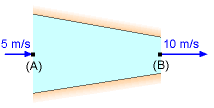Problem Description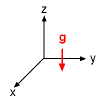Sign Convention for the Gravitational Acceleration (a) For steady flow, Euler's equation in vector form is given by: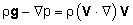If one assumes the z-axis is positive upward, then the gravitational acceleration g can be written as (see figure):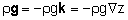Now substitute the vector identity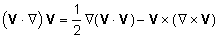to write Euler's equation as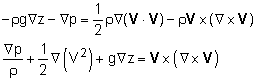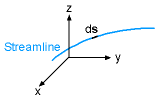Differential Length on a Streamline In order to obtain Bernoulli's equation, one needs to integrate the above equation along a streamline. Hence, take the dot product of Euler's equation with a differential length ds along the streamline as follows: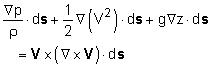Recognize that the differential length ds can be expressed in terms of the Cartesian coordinates as      ds = dx i + dy j + dz k Each term on the left hand side of Euler's equation can be evaluated as follows: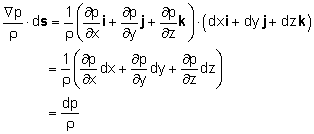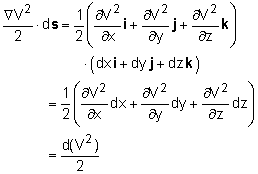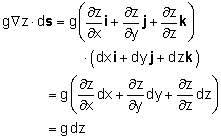Since streamlines are constructed such that they are tangent to the velocity field, the differential length ds is parallel to the velocity V. The vector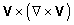is then perpendicular to V, which gives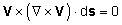Euler's equation thus becomes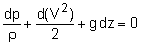which can be readily integrated to yield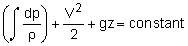For constant density, the above equation reduces to the famous Bernoulli's equation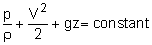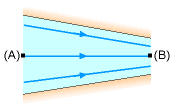Streamline between Point A and B (b) Apply Bernoulli's equation between the inlet and outlet of the nozzle (i.e., along the streamline between points A and B),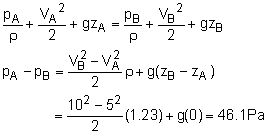Practice Homework and Test problems now available in the 'Eng Fluids' mobile app
Includes over 250 problems with complete detailed solutions.
Available now at the Google Play Store and Apple App Store.